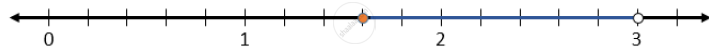# Solve the Following Inequation, Write the Solution Set and Represent It on the Number Line -x/3 <= X/2 - 1 1/3 < 1/6, X ∈ R - Mathematics

Solve the following inequation, write the solution set and represent it on the number line -x/3 <= x/2 - 1 1/3 < 1/6, x ∈ R

#### Solution

The given inequation is -1/3 <= x/2 - 1  1/3 < 1/6, x ∈ R

-x/3 <= x/2 - 1 1/3

x/3 - x/2 <= -4/3

(2x + 3x)/6 >= 4/3

(5x)/6 >= 4/3

5x >= 8

x >= 8

x >= 8/5

x >= 1.6

and

x/2 - 1 1/3 < 1/6

x/2 < 1/6 + 4/3

x/2 < (1+8)/6

x/2 < 9/6

x < 18/6

x < 3

The solution set is {x: 1.6 <= x < 3, x ∈ R}

It can be represented on a number line as follows:Concept: Representation of Solution on the Number Line
Is there an error in this question or solution?